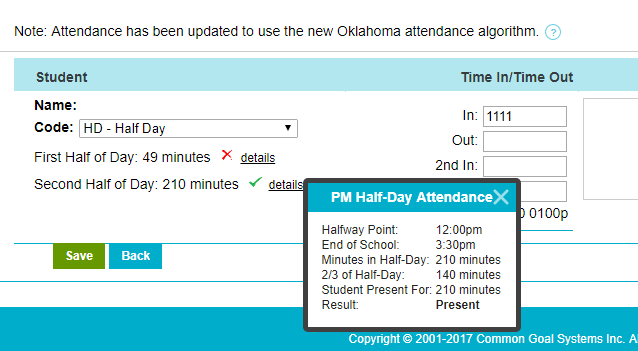# How is Oklahoma attendance calculated?

In the state of Oklahoma, a student is considered present for the whole day if they have been present for at least ⅔ of both the AM and PM half of the day.  AM Half-Day is marked by the start of the day until the halfway point; whereas, the PM Half-Day starts at the halfway point and lasts until the end of the school day.  The halfway point on any school day can be calculated by dividing the total number of minutes in the school day in half. This article explains how SchoolInsight accommodates this mode of calculating the daily attendance as defined by the WAVE for Oklahoma schools.For example, if a school's instructional day begins at 8:00 am and ends at 3:15 pm, this means that the school day is 7 hours and 15 minutes long, or it has 435 total minutes.  435 minutes divided in half is 217.5 minutes.  As a result, the halfway point of that school's instructional day would be 217.5 minutes from 8:00 am, or between 11:37 am and 11:38 am.

To be counted as in attendance for the AM Half-Day, a student would need to be present for 145 minutes (2/3 of 217.5 minutes) between 8:00 am and 11:37 am.  To be counted in attendance for the second half of the day, the student would need to be present for 145 minutes between 11:38 am and 3:15 pm.

Following the example given above, here are few interesting cases that explain how a student's attendance code is calculated:

• If a student is present for at least 145 minutes in the AM Half-Day as well as in the PM Half-Day, then that student would receive a present attendance code for the day.
• If a student is present for at least 145 minutes only in the AM Half-Day or only in the PM Half-Day, then that student would receive a half-day present code only.
• If a student comes at 10:00 AM and leaves the school at 1:00 PM, then that student would receive an absence for the day.  In spite of being in the school for 180 minutes, this student was present for only 97 minutes (from 10:00 AM to 11:37 AM) in the AM Half-Day, and the student was present for only  82 minutes (from 11:38 AM to 1:00 PM) in the PM Half-Day. In both cases the student was present less than ⅔ of the AM Half-Day and the PM Half-Day; therefore, the student is considered absent for the whole day.